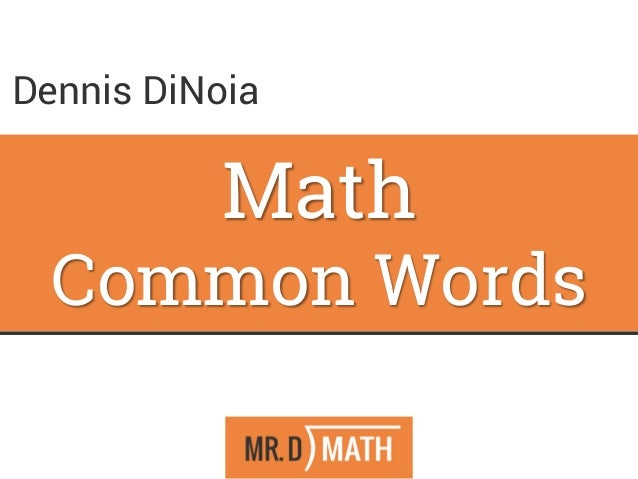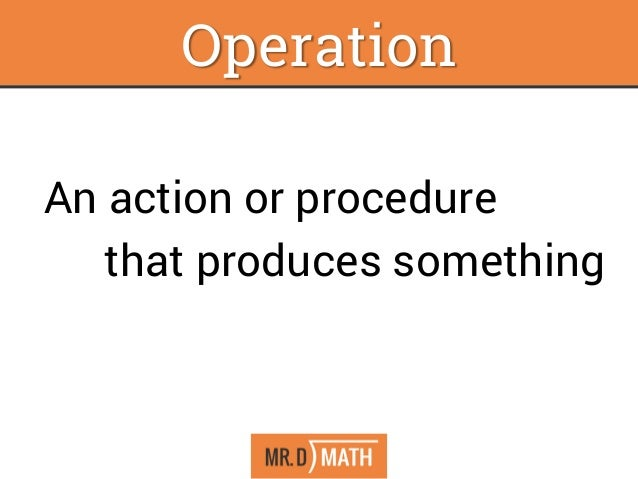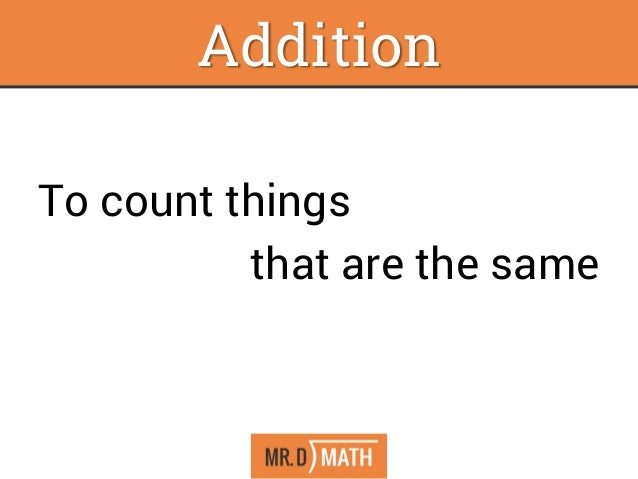Successfully reported this slideshow.Upcoming SlideShare
×

ofUpcoming SlideShare
Next

0

Share

# How To Think And Talk Math

What if math was its own language? Learn to speak math similar to the way you learn to speak other new languages. Learn the basic foundations on which the math language is created and how to see Algebra skills in basic math. This workshop will give participants wanting to expand their thinking to higher levels of math using basic operations they already know. Learn how the “secrets” of mental math and how to apply them in everyday situations. Participants access a common math language that can be used at any grade level.

See all

### Related Audiobooks

#### Free with a 30 day trial from Scribd

See all
• Be the first to like this

### How To Think And Talk Math

1. 1. Math Common Words Dennis DiNoia
2. 2. The Tools of Algebra Section 1.3 An action or procedure that produces something Operation
3. 3. The Tools of Algebra Section 1.3 To count things that are the same Addition
4. 4. The Tools of Algebra Section 1.3 Add Bigger Ascend Plus Grow Deposit More Up Increase Total Positive Greater Sum Words That Mean “Add”
5. 5. The Tools of Algebra Section 1.3 1) A way to find out how far it is between things (distance) 2) To take from something that is already there Subtraction
6. 6. The Tools of Algebra Section 1.3 Subtract Descend Smaller Minus Take away Withdraw Less Down Decrease Negative Difference Words That Mean “Subtract”
7. 7. The Tools of Algebra Section 1.3 To count things by more than one at a time Multiplication
8. 8. The Tools of Algebra Section 1.3 Multiply Times By Of Product Words That Mean “Multiply”
9. 9. The Tools of Algebra Section 1.3 To separate things into equal groups *A remainder is what is left over if it does not fit into one of the equal groups. Division
10. 10. The Tools of Algebra Section 1.3 Divide Separate Cut Ratio Per Quotient Words That Mean “Divide”
11. 11. The Tools of Algebra Section 1.3 Per means Divide Cent is a prefix for 100 (as in Century) Percent means divide by 100 Percent
12. 12. The Tools of Algebra Section 1.3 Equal Is The Same As Equivalent Congruent Words That Mean “Equal To”
13. 13. The Tools of Algebra Section 1.3 Things being equal in time … Is – Happening now Was – Happening before Will be – Happening in the future Words That Mean “Equal To”
14. 14. The Tools of Algebra Section 1.3 Is Less Than < Is Less Than or Equal to < At Most < Is Greater Than > Is Greater Than or Equal to > At Least > Words That Mean “Not Equal To”

Total views

20,083

On Slideshare

0

From embeds

0

Number of embeds

19,840

3

Shares

0